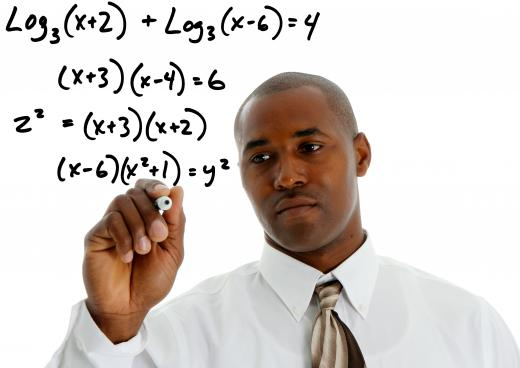# What is the Order of Operations?Mary McMahonMary McMahonThe order of operations is a set of rules which must be kept in mind when doing math problems.

The order of operations is a set of rules which must be kept in mind when doing math problems. These rules tell people when to perform various operations in a math problem with mixed operations, such as (7 + 2) x 4 - 3. There are a number of possible answers to this problem, depending on the order in which the multiplication, subtraction, and addition are performed, but only one right answer, because the order of operations tells people how to do the problem.Expanding logarithms by obeying the order of operations enable equations to be solved.

According to the order of operations, when one is faced with a math problem which has mixed operations, anything in parentheses should be done first, followed by exponents and roots, and then, working from left to right, multiplication and division. Finally, also working from left to right, addition and subtraction. People sometimes use the acronym PEMDAS, for Parentheses, Exponents, Multiplication, Division, Addition, and Subtraction, to remember the order of operations. The mnemonic "please excuse my dear Aunt Sally" to help people learn this acronym is used in a number of beginning math classes.

Taking the problem in the example above, the first thing to do would be the addition inside the parenthesis, 7+2, which equals 9. Next, the multiplication should be done, to reach 36. Finally, the 3 must be subtracted, for a total of 33. The order of operations applies to any math problem, from simple to complex. If there weren't a particular order established, people could come up with equally correct results. For example, someone could read the above problem and come up with an answer of 9, by adding 7+2 to get 9, subtracting 3 from 4 to get 1, and multiplying 9 by 1 to arrive at 9.

The left to right rule for addition and subtraction and multiplication and division in the order of operations is also important. In a problem like 9 - 7 + (4 x 5) ÷ 10, for example, one would do the parenthesis first, ending up with 9 - 7 + 20 ÷ 10. Division comes next, so 20 ÷ 10 = 2. Addition doesn't take precedence over subtraction, so these are done left to right. The answer to the problem is therefore 4, because 9 - 7 = 2, and 2 + 2 = 4. Prioritizing addition over subtraction and not following the left to right rule would result in 9 - 9 = 0, a very different answer!

In a way, the order of operations tells people how to read math problems, just like the rules of grammar tell people how to read written languages. The rules of grammar and mathematics are both designed to make sure that everyone can write and read in a universal way which ensures that people can communicate freely with people they may never personally interact with. The standardization created by the order of operations is especially important in mathematics because there are so many ways to work complex problems without it, and this would result in a multitude of conflicting answers.Mary McMahon

Ever since she began contributing to the site several years ago, Mary has embraced the exciting challenge of being a AllTheScience researcher and writer. Mary has a liberal arts degree from Goddard College and spends her free time reading, cooking, and exploring the great outdoors.Mary McMahon

Ever since she began contributing to the site several years ago, Mary has embraced the exciting challenge of being a AllTheScience researcher and writer. Mary has a liberal arts degree from Goddard College and spends her free time reading, cooking, and exploring the great outdoors.